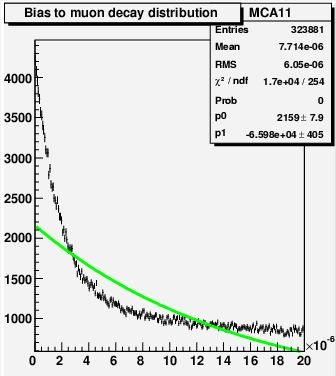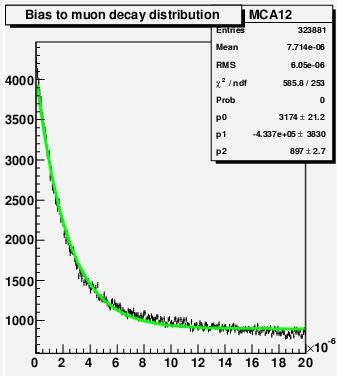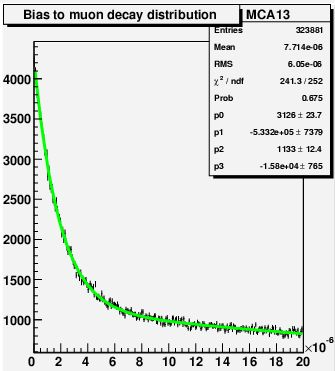## Answer to the muon decay experiment questionJune 5, 2008

Posted by dorigo in personal, physics, science.
Tags: , , , ,

Ok, one day has passed, and a few readers have decided to leave a comment with their answer to the question I posed in the former post, along with some additional considerations. I will first show the answer to the question pictorially, by pasting here four graphs I obtained this evening with a simple root macro. The macro perform a simple simulation of the experiment and -save some (unlikely) mistake on my part, it allows to obtain a histogram of counts in the 256 bins of a simulated multichannel analyzer (MCA), mapping the time frame$0 - 20 \mu s$ after a start has been detected by the experimental setup. The macro also allows the user to vary the number of bins of the MCA, the fraction of background, the exposure -but we need not be concerned with that.

A word of warning: the simulation is crude – I did not bother to insert the geometry of the apparatus (which may change from one setup to another), the energy loss of muons and electrons in Aluminum, or other details: I just simulated at random muon decays and background, recording whichever produces a stop in the MCA counts. Also, note that only positive muons are simulated here: as I warned in the post-scriptum to my post of yesterday, and as is further discussed in the thread to that post, negative muons get captured by Aluminum nuclei in the K-shell, and they decay much faster than positive ones -about 2.5 times more so. The decay produces a nuclear recoil which gets recorded in the scintillator as if it were an electron, causing in the end the MCA to record not one, but two different exponential distributions (with time constants of 2.2 and 0.88 microseconds for positive and negative muons, respectively) on top of random backgrounds. I ask the reader to forget about this detail in the following.In the plots you see the stop time distribution resulting from a million starts, in a situation when there is a large amount of background, and when a realistic fraction of positrons get detected by the apparatus (roughly 30%). The first plot shows the MCA distribution fit with a single exponential (answer #1). This is a very wrong modeling of what is happening, of course: in the presence of background -caused by particles randomly crossing scintillators B or C during the 20 microseconds of exposure after a real muon start- the multichannel will record both a muon decay signal with a time structure, and casual hits at random times.

The second plot shows how the data is interpreted with a functional form representing answer #2: a falling exponential plus a constant. Here things look definitely better, but a keen eye will spot the problem in the fit: the green curve stays above, then below, and then above the data points as we move left to right. In fact, in the upper right corner root informs us that the fit probability is zero! There is thus something wrong with answer #2.The third plot shows how the data is interpreted with a functional form representing answers #3 and #4: two falling exponentials with different time constants. The fit now is extremely good! The fit probability is a whooping 67.5%, indicating that our model of the data is correct, within the statistical power of the analyzed data. What makes options #3 and #4 different is that in the former case one expects one of the two exponentials to have a decay constant equal to the muon lifetime -which in the simulation was set to 2.0 microseconds for simplicity, while option #4 predicts that the lifetime measurement will be biased. Well, by looking at the parameters of the fitting function (which is$p_0 e^{p_1 t} + p_2 e^{p_3 t}$) one observes that neither$p_1$ nor$p_3$ have a value close to$-1/0.000002=-500,000$ (remember, the decay law is$e^{-t/\tau_\mu}$ so the fit parameter describing the exponential slope has to be the inverse of the opposite of the muon lifetime).

The fourth plot -at this point, only academic- shows the results of a constant fit. Of course, there is a lot of time dependence in the data, and not just a flat distribution!Now let me comment the result. Why are we finding that the background -which is uncorrelated with the time of arrival of stopped muons- shows a time structure ? And why on earth is the muon lifetime coming out biased low (the fit in the third plot has a value$p_0$ larger than 500,000) ?

The answer is not quite simple, but one can arrive at it by intuition, as I mentioned in the former post. First of all, we have to note that while the muon causing the start of our clock has one and only one chance to yield a signal -with the exponential law we discussed above-, backgrounds, however small, have multiple, albeit tiny, chances of providing counts in the time window: only the first arriving background count stops our clock and records an entry in the MCA, though. So, imagine you have not just one (and in our experimental setup, there is only a 20% chance that a background hit occurs in the 20 microseconds), but many background hits distributed at random throughout your 20 microseconds time window: they are overall flat -no time information from them- and yet, our data acquisition system records the first one! No wonder we get an exponential for the background as well!

So, the background produces an exponentially falling distribution, as showed by the fit in picture three. But why, however, do we get a wrong lifetime from the hits really coming from muon decays ?

The reason is the same as above. Muons decaying early on have a smaller chance of being beaten on time by a random background hit than muons decaying later on. So, the time distribution of real muon decay stops is also biased towards smaller values. We get a time constant smaller than the muon decay lifetime, that is.

Not convinced ? I can provide the root macro, and (by downloading the root package from cern) you should be able to test the system by yourself in minutes. Drop me a line if you wish to.